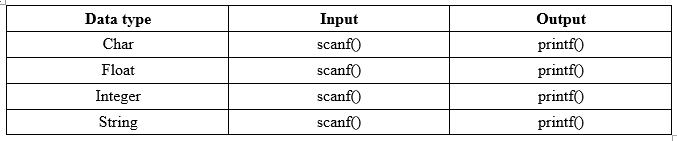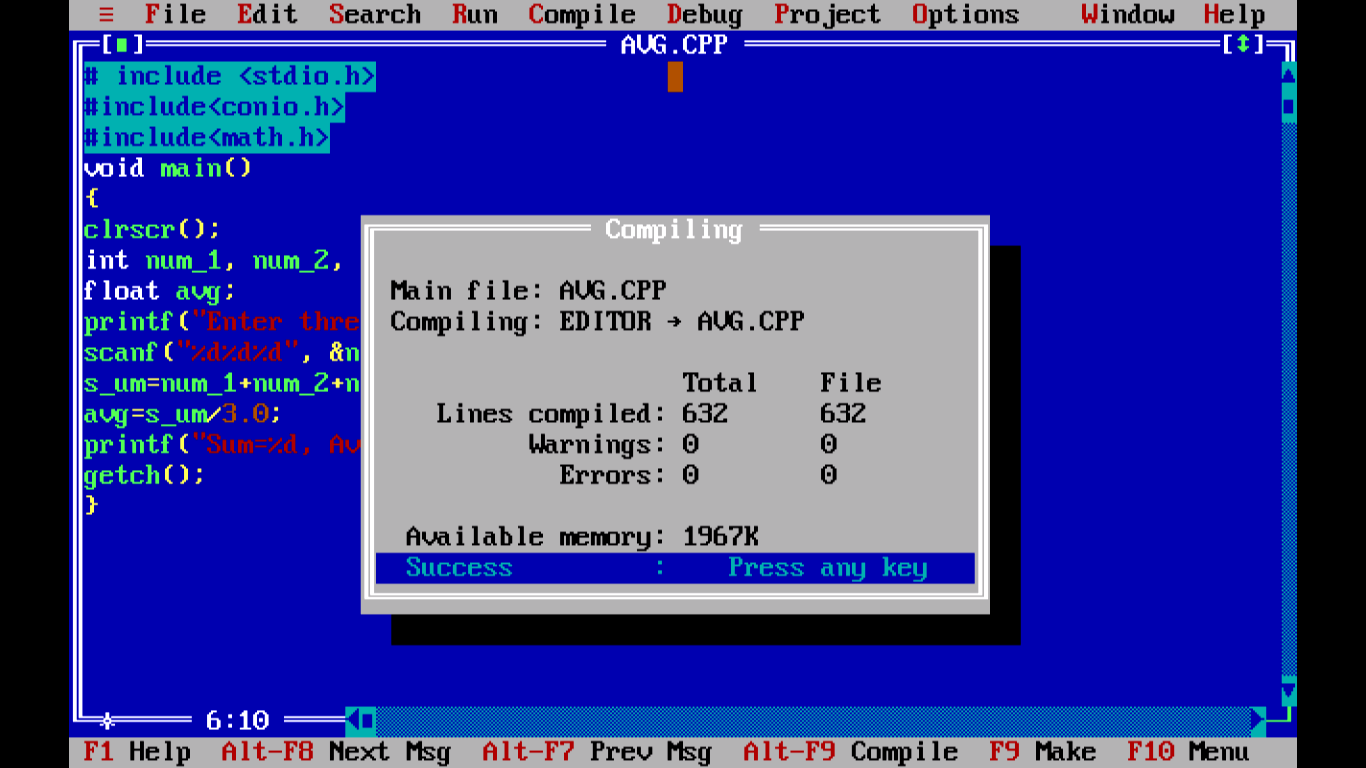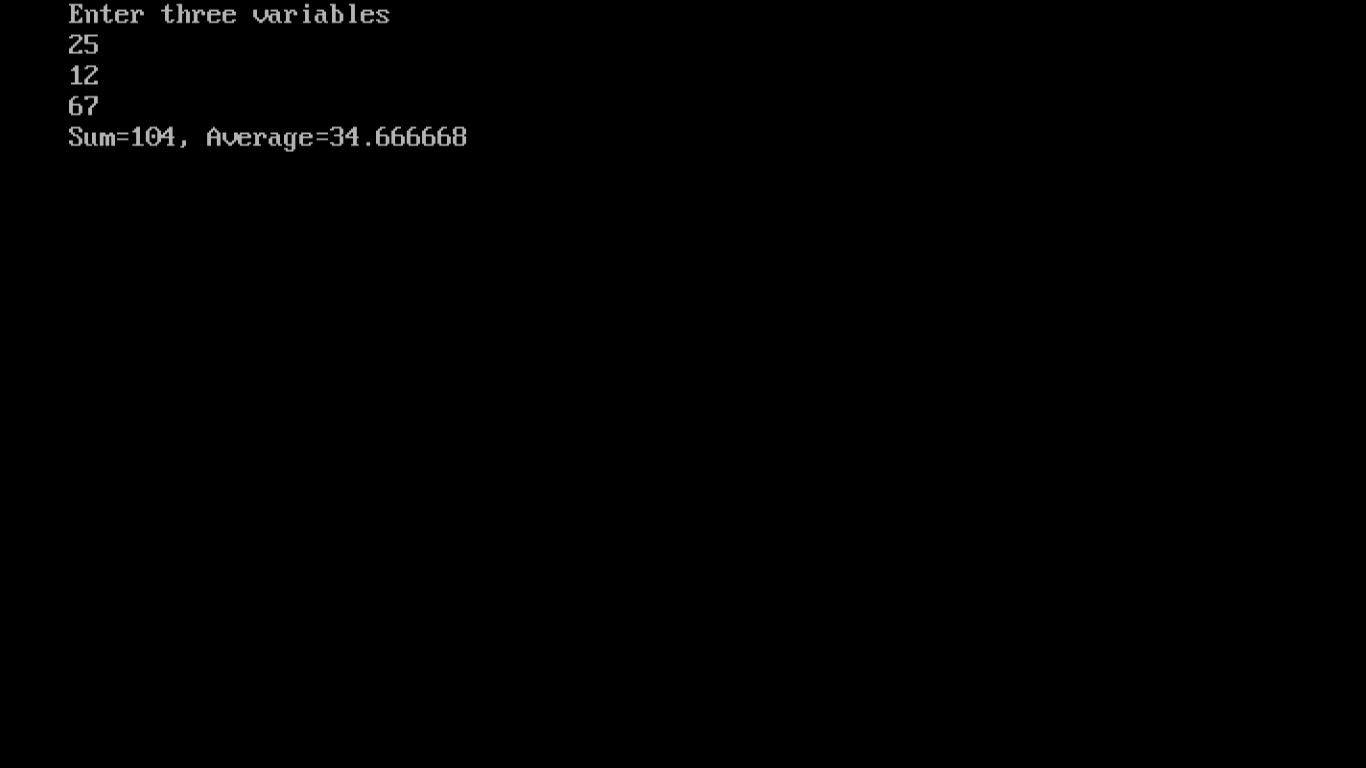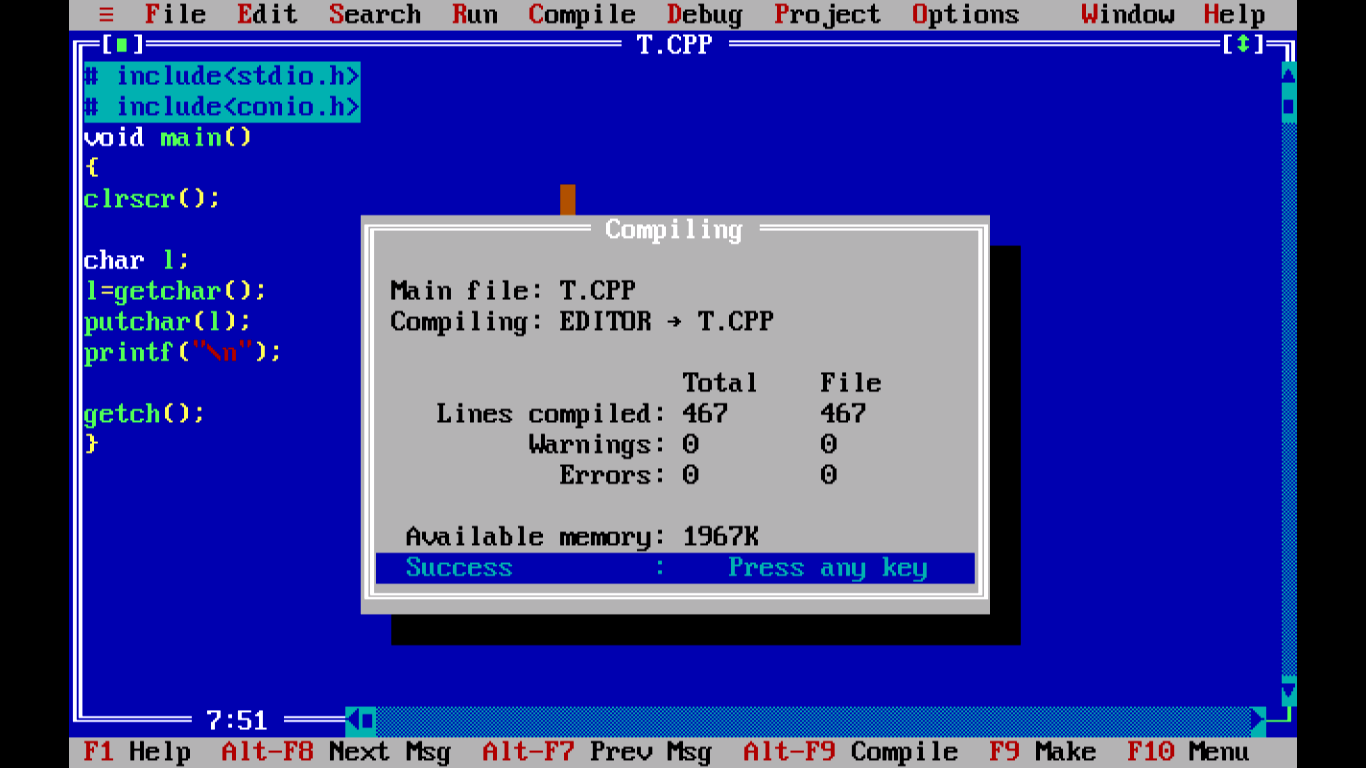# 12 - Input / Output in C

In this chapter we shall discuss the input/output functions or features in C programming language. Did you ever wonder, what we type in the keyboard while writing a C program is accepted or we can see it on monitor and how this background process is going on? Today we shall deal with this. We shall see the input/output functions in C. C is known as functional programming language and hence there is no in-built library function exclusively which does input/output. There are lot of library functions which deal with input / output. These can be broadly categorized as:

1.Console I/O functions

2.Disk I/O functions

3.Port I/O functions

Console I/O functions: These functions are responsible to receive input from keyboard and write  output to Video display unit (monitor).

Disk I/O functions: These functions are responsible for processing input and output functions on hard  disk or floppy drives.

Port I/O functions: These functions are responsible for carrying input and output operations on  different ports.

We shall cover Console Input output functions

## 12.1 Console Input output functions

The library functions is known as standard input-output library. The corresponding header file is stdio.h . There are two types of input/ouput functions namely:

• Formatted input/output function
• Unformatted input/output functions

The following diagram would explain and give a quick glance of description we are going to cover under Formatted Console Input output functionsFormatted Console Input output functions

## 12.1.1Formatted Input Function

Folks! You have already used this function unknowingly and this is scanf() function. This function is responsible to input values for variables from keyboard. The scanf() function is to used to input numeric, string and character data type. Format of this function is as follows:

Explanation: Here,

string of control: Sequence of character groups is entered here. This is usually a combination of one or more conversion characters following % symbol and this describes types of values which are to be specified to the variables.

address: This is a list of addresses which represents the address of memory locations  where the values we specify for input is stored.

We shall summarize all the different character groups in the following table so that it becomes easy for you to become familiar with these symbols.Table: Summary of  C potential character groups

Examples of formatted input functions

1)Interger Input : We input decimal integer data via standard input device using %d character and following example illustrates the usage of integer type

Example: scanf(“%d”, &anynumber);

Explanation: In the above scenario, whatever value you input from standard input, say 10, is going to be stored in a memory location which is represented by anynumber.

Example: scanf(“%4d”, &number);

Explanation: There is a procedure which is known as Field specification. As the term indicates, it is  the way of reserving number of fields for corresponding input. In the above scenario we are introduced to a different type of way to input data through standard input device. %d  is the conversion character and number 4 is known as field width. The number of characters in data valiue should not exceed the specified field width. In case, you exceed the specified field width then that extra value is not going to stored in memory. We can input any four digit number 1234, 5678, etc.

2)Character Input : We input character group using character constant using %c conversion character. The string constant requires %s conversion character.

Exampe: scanf(“%c”, &rep) ;

Explanation: In the above example, rep represents a variable of type character. For instance we input A then corresponding ASCII value associated with this symbol is stored in the memory  location and in this example it is 65 as ASCII value corresponding to A is 65.

Example: scanf(“%5c”, myname);

Explanation: In the above scenario, we do not have an ampersand symbol. Here we specify the total  number of characters present in the input or the address is to be specified. As we do not have an ampersand symbol so prior to decalring this particular statement we also need to declare a variable name myname as one dimensional character array before we expect it  to accept the input so the array should be declared as follows:

char myname;

Example: scanf(“%s”, mynewname) ;

Explanation: In the above scenario, we  are showing the way to input string constant. As you can see  there is no (&) ampersand symbol so we have to declare mynewname as a one  dimensional character array.

3)Floating point Input : We input floating point numbers by using %f conversion character and in contrast to integer type we usually do not specify the field width for real numbers.

Example: scanf(“%f”, &z);

Explanation: In the above scenario, if we input 55 then vale 55.0 value is stored in the memory location specified by z.

Example: scanf(“%lf”, &n);

Explanation: In the above scenario, we target to input a double precision number. Here the data type  type id double so we use %lf instead of %f.

Let us move to formatted output.

## 12.1.2Formatted Output Fnction

Formatted output function is printf()function. This function displays data on statndard output and that is monitor and this function is included in stdio.h header file. Format of this function is as follows:

printf(“string of control”, list_of_variables);

Explanation: Here,

string of control: This specifies the data type and format of values which are going to be  displayed on standard output

list_of_variables: This specifies list of variables associated with values meant to be  displayed on monitor

Examples of formatted output functions

1)Integer type: We use %d conversion character to display the integer type data on standard output. It is similar to formatted input but scanf is replaced by printf and there is no (&) ampersand symbol.

Example: printf(“%d”, number);

Explanation: In the above scenario, variable number is to be displayed on standard output. For   instance its value is 5 then number 5 is displayed on monitor as output.

2)Character type: This is meant to display character or character group also known as string. We need the same printf function.

Example: scanf(“%c”, character);

Explanation: In the above scenario, character variable is of char type data. Now whatever, we enter as input that is going to appear on screen. For example if we enter B as input then we will see B as output after executing the above statement.

Example: printf(“%s”, name);

Explanation: In the above example, we will have a string as ouput which is represented by variable name of type string. In this scenario we will have to declare this variable name before using it say for instance

char  name;

3)Floating point type: Floating point number is displayed either in decimal form or scientific notation. Usually we use %f as conversion character.

Example: printf(“%f”, z);

Explanation: In the above scenario, if variable z stores 25 then 25.000000 will be displayed on screen.

Example: printf(“%7.2f”,z);

Explanation: In the above scenario, we can have control over the number of digits after decimal point by the usage of field width specifier. Above instance is going to be interpreted as we are going to print a  number of maximum 7 characters which includes decimal point and there should be two digits after decimal point. For example, if we have 243.1234 as the  target to be displayed or variable z has this number then after executing the above  statement, we will have 243.12 as otput on monitor.

/* C Program to illustrate formatted input and output functions*/

```# include<stdio.h>
#include<conio.h>
#include<math.h>

void main()
{
int num_1, num_2, num_3, s_um;
float avg;

printf("Enter three numbers\n");
scanf("%d%d%d", &num_1, num_2, num_3, s_um;
s_um=num_1+num_2+num_3;
avg=s_um/3.0;
printf("Sum=%d, Average=%f", s_um, avg);
getch();
}
```Figure - Sample C Program illustrate formatted input and output functionsCompiled output of Sample C Program illustrate formatted input and output functionsLet us proceed with unformatted input and output functions

## 12.1.2Unformatted Input Function

These functions read character type data from keyboard and we have two functions namely:

• getchar()
• gets()

And both of these functions are included in stdio.h header file.

1. The getchar() function: This function is responsible for reading only one character from standard  input. We do not need parameter within the brackets.

Format: character=getchar();

Explanation: Here,

character is a char type of data variable and it accepts the character assigned.

2. The gets() function: This function is responsible for accepting input from keyboard and continues to   accept input until enter key or return key is pressed. Whatever we type is stored   as string which in turn in sequence of ASCII characters which are printable except the Enter key which we press in the end.

Format: gets(str);

Explanation: Here,

str represents sequence of characters which is of char data type

Let us have a quick glance of unformatted output.

## 12.1.3Unformatted Output Function

These functions will print vales of character data type on monitor. We have two functions and they are also stored in stdio.h header file:

• The putchar() function
• The puts() function

Let us have a glance on this topic as well.

1. The putchar() function: This function is responsible for printing only one character on monitor and the supported data type is char.

Format: putchar(character);

Explanation: Here,

character is a char data type variable

2. The puts() function: This function prints sequence of characters on monitor. The end of string is  newline character and it is not displayed on monitor.

Format: puts(str);

Explanation: Here,

str represents string of characters

/*Program to illustrate unformatted input output functions*/

```# include <stdio.h>
# include <conio.h>

void main()
{
char l;
l=getchar();
putchar(l);
getch();
}
```Figure - C Program to illustrate unformatted input output functionsFigure - Compiled output of  C Program to illustrate unformatted input output functionsFigure - output of  C Program to illustrate unformatted input output functions

Folks! We are good to go now. With this we conclude this chapter and next chapter will introduce Arrays. Thank you.